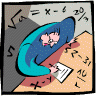## Mathematics, Department of## Dissertations, Theses, and Student Research Papers in Mathematics

L. L. Avramov

S. B. Iyengar

8-2017

#### Comments

A DISSERTATION Presented to the Faculty of The Graduate College at the University of Nebraska In Partial Fulfillment of Requirements For the Degree of Doctor of Philosophy Major: Mathematics Under the Supervision of Professors L. L. Avramov and S. B. Iyengar Lincoln, Nebraska August, 2017

Copyright (c) 2017 Luigi Ferraro

#### Abstract

This thesis consists of two parts:

1) A bimodule structure on the bounded cohomology of a local ring (Chapter 1),

2) Modules of infinite regularity over graded commutative rings (Chapter 2).

Chapter 1 deals with the structure of stable cohomology and bounded cohomology. Stable cohomology is a \$\mathbb{Z}\$-graded algebra generalizing Tate cohomology and first defined by Pierre Vogel. It is connected to absolute cohomology and bounded cohomology. We investigate the structure of the bounded cohomology as a graded bimodule. We use the information on the bimodule structure of bounded cohomology to study the stable cohomology algebra as a trivial extension algebra and to study its commutativity.

In Chapter 2 it is proved that if a graded, commutative algebra \$R\$ over a field \$k\$ is not Koszul, then the nonzero modules \$\mathfrak{m} M\$, where \$M\$ is a finitely generated \$R\$-module and \$\mathfrak{m}\$ is the maximal homogeneous ideal of \$R\$, have infinite Castelnuovo-Mumford regularity.

Advisers: L. L. Avramov and S. B. Iyengar

COinS